Ph - C $\equiv \mathrm{CH}\underset{\mathrm{MeOH}}{\overset{{\mathrm{MeO}}^{-}}{\to }}$ Major product of the reaction is:

(a)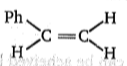(b)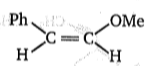(c) Ph - C $\equiv$C-OMe

(d)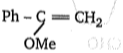High Yielding Test Series + Question Bank - NEET 2020

Difficulty Level:

; Product (A) is:

(a) Ph-CH=CH2          (b) Ph-C$\equiv$CH              (c) Ph-CH2-CH3             (d) Ph-C$\equiv$$\stackrel{⊝}{\mathrm{C}}\stackrel{\oplus }{\mathrm{Na}}$

High Yielding Test Series + Question Bank - NEET 2020

Difficulty Level: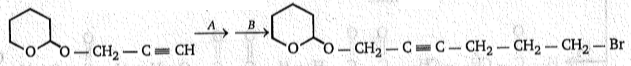To carry out above conversion, (A) and (B) respectively, are :

(a) NaNH2, Cl - CH2ーCH- CH- Br
(b) NaNH2, CH2-CH2-CH2-Br
(c) NaNH2, l-CH2ーCH2-CH2-Br
(d) NaNH2, I-CH2-CH2-CH2-I

High Yielding Test Series + Question Bank - NEET 2020

Difficulty Level:

H-C$\equiv$C-Ph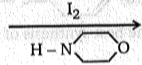Product; Product obtained in this reaction is:

(a) $\begin{array}{ccccccc}\mathrm{Ph}& -& \underset{\mathrm{I}}{\underset{|}{\mathrm{C}}}& =& \mathrm{CH}& -& \begin{array}{c}\mathrm{I}\end{array}\end{array}$

(b) $\begin{array}{ccccccc}\mathrm{PH}& -& \underset{\mathrm{I}}{\underset{|}{\mathrm{CH}}}& -& {\mathrm{CH}}_{2}& -& \mathrm{i}\end{array}$

(c) Ph-C$\equiv$C-I

(d) I-C$\equiv$C-H

High Yielding Test Series + Question Bank - NEET 2020

Difficulty Level: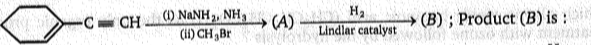(a)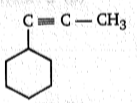(b)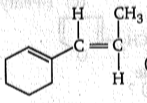(c)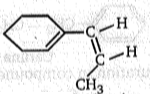(d)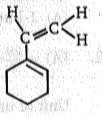High Yielding Test Series + Question Bank - NEET 2020

Difficulty Level:

Which of the following alkyne on treatment with H2(2 mole)/ Pt gives an optically inactive compound ?

(a) 3-Methyl-1-pentyne

(b) 4-Methyl-1-hexyne

(c) 3-Methyl-1-heptyne

(d) None of the above

High Yielding Test Series + Question Bank - NEET 2020

Difficulty Level: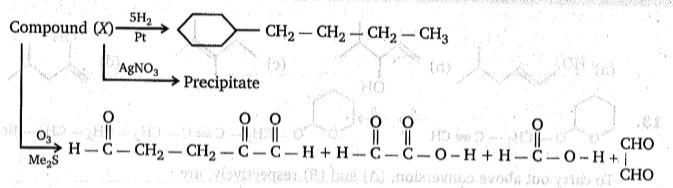Compound (x) will be:

(a)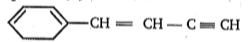(b)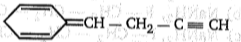(c)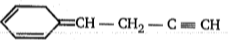(d)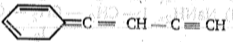High Yielding Test Series + Question Bank - NEET 2020

Difficulty Level:

Which alkyne yields butanoic acid (CH3CH2CH2CO2H) as the only organic product on treatment with ozone followed by the hydrolysis?

(a) 1-Butyne             (b) 4-Octyne                (c) 1-Pentyne                (d) 2-Hexyne

High Yielding Test Series + Question Bank - NEET 2020

Difficulty Level: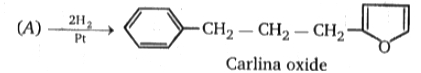Unit of unsaturation in compound (A)?

(a) 7                         (b) 8                        (c) 9                             (d) 10

High Yielding Test Series + Question Bank - NEET 2020

Difficulty Level: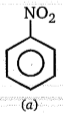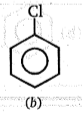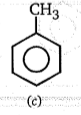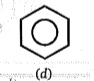Correct order of rate of EAS (electrophilic aromatic substitution) is :

(a) c > b > a > d

(b) c > d > a > b

(c) a > b > c > d

(d) c> d> b > a